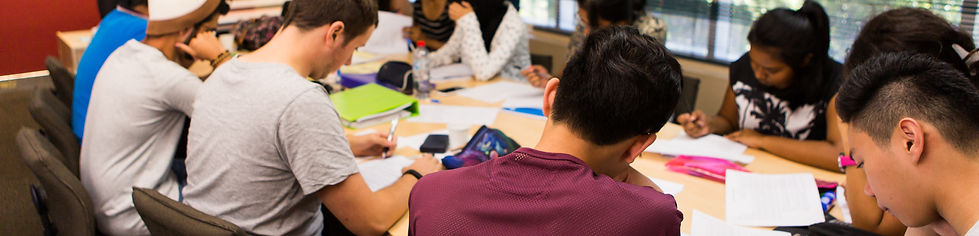Year 12 HSC
Mathematics (General)

By the end of Year 12, students will be able to use two-dimensional and three-dimensional models to solve practical problems and understand the relationship between changing quantities in algebraic and graphical form. Students will be able to solve and apply Pythagoras’ theorem and trigonometric ratios to trigonometric problems. They will be able to model financial situations and represent collected data appropriately. Students will be able to use statistics and analyse data to make inferences, predictions and conclusions. They will be able to make predictions based on mathematical models and about financial situations. Students can use results of measurements and calculations to make judgements about reasonableness. Students can apply mathematical techniques to real life through mathematical argument and reasoning.

Timetable